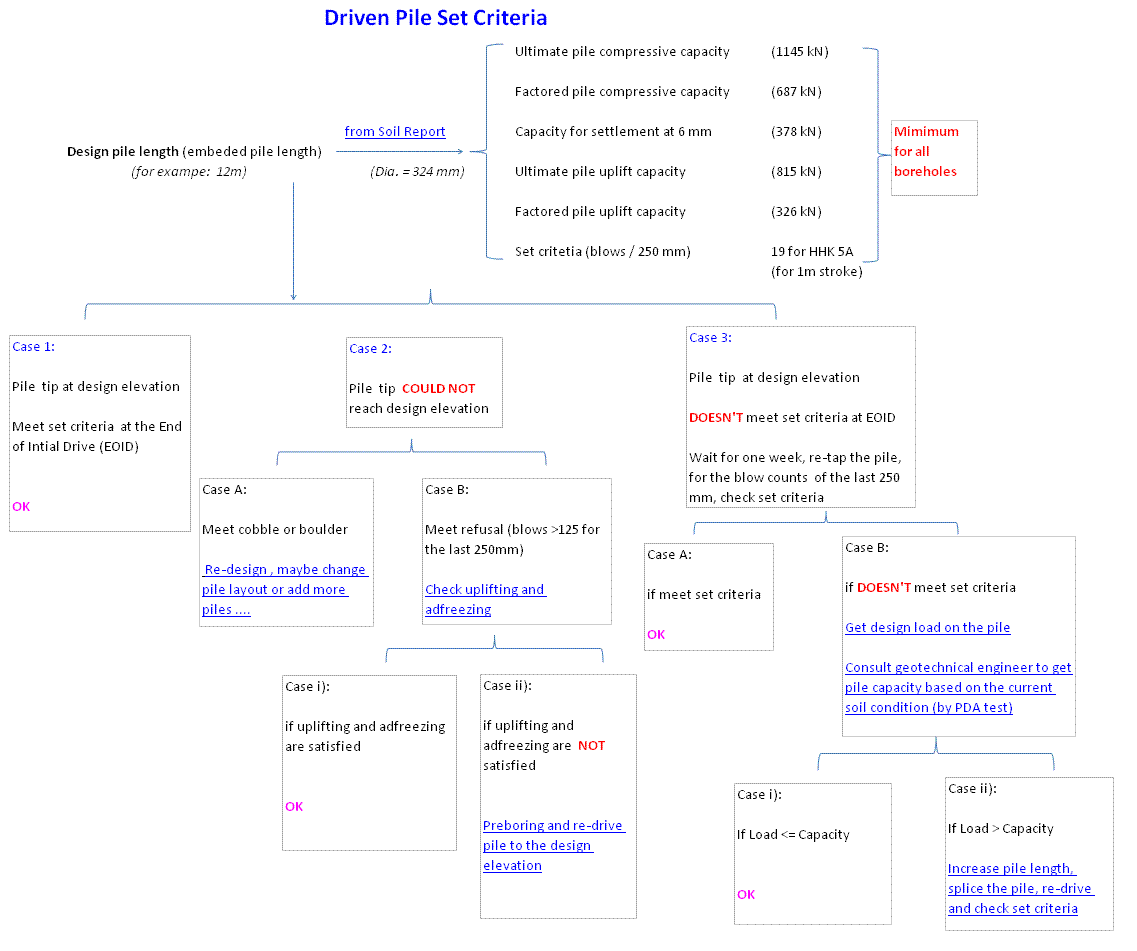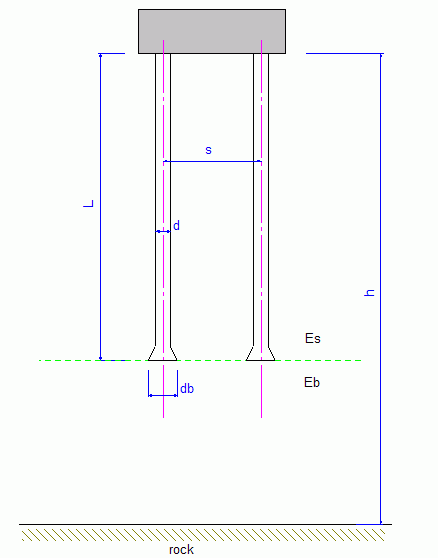HomeGeneral Tools
-------------------------------------------------------------------------------------------------------------------------------------------------------------------------------------------------------------------------------------------
Group factor of pile settlement
h = m Pile Information & Layout Ny = m Nx = Sx = Sy = m d = m L = m K = m db =Group Factor of Pile SettlementGroup Factor Rs =
Pile Cap
Soil Information u =Assumption:
Equal settlement of all piles, corresponding to a perfectly rigid cap on the piles.

The following factors are incoporated in the analysis:
Factor of finite layer: Nh, normally, set h > 5L, Nh = 1.0
Factor of enlarged pile base: Ndb
Factor of poisson's ratio: Nv
Factor of linearly increasing modulus of elasticity: Nes
Factor of finite compressibility of bearing stratum: NFE, for end-bearing piles only

The shaft friction is dominant of the pile capacity for floating piles.

The pile end bearing is dominant of the pile capacity for the end-bearing piles.

Eb is modulus of elasticity of soil under the pile tip, Es is average modulus of elasticity of soil along the pile shaft. factor of Eb/Esis only applicable to end-bearing piles with soils between the pile tip and rock.

Pile stiffness factor: K = Ep.RA / Es
Ep - modulus of elasticity of the piles
Es - average modulus of elasticity of soil along the pile shaft
RA = Ap / (0.785 d2), Ap - the area of pile cross section, for solid pile RA = 1

The basic equation for the analysis:
rk = S(r1j .Pj.akj) + r1k.Pk

r1j = displacement of single pile j under unit load
rk = final displacement of single pile k
akj = interaction factor for spacing between piles k and j, and for the geometrical parameters of pile j